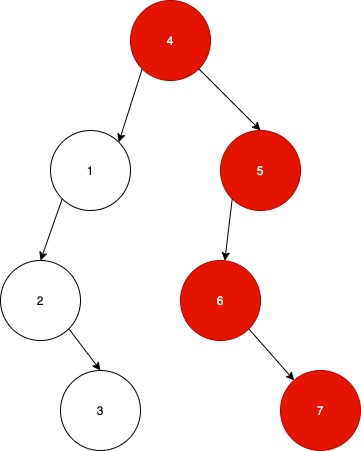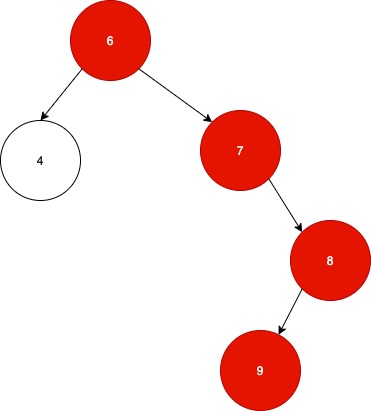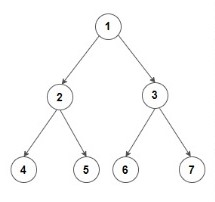1

# Longest Consecutive Sequence in a Binary Tree

Difficulty: EASY
Avg. time to solve
10 min
Success Rate
90%

Problem Statement
Suggest Edit

#### For example:#### Note that there is another consecutive sequence of length 3 i.e. 1->2->3 in the left subtree of the root node, but it is not the longest consecutive path.

##### Note:
``````1. Do not print anything, just return the length of the longest consecutive path in the binary tree.
``````
##### Input Format:
``````The first line contains an Integer 't' which denotes the number of test cases or queries to be run. Then the test cases follow.

The first line of input contains the elements of the tree in the level order form separated by a single space.
If any node does not have left or right child, take -1 in its place. Refer to the example below.

Example:

Elements are in the level order form. The input consists of values of nodes separated by a single space in a single line. In case a node is null, we take -1 on its place.

For example, the input for the tree depicted in the below image would be :
````````````1
2 3
4 -1 5 6
-1 7 -1 -1 -1 -1
-1 -1

Explanation :
Level 1 :
The root node of the tree is 1

Level 2 :
Left child of 1 = 2
Right child of 1 = 3

Level 3 :
Left child of 2 = 4
Right child of 2 = null (-1)
Left child of 3 = 5
Right child of 3 = 6

Level 4 :
Left child of 4 = null (-1)
Right child of 4 = 7
Left child of 5 = null (-1)
Right child of 5 = null (-1)
Left child of 6 = null (-1)
Right child of 6 = null (-1)

Level 5 :
Left child of 7 = null (-1)
Right child of 7 = null (-1)

The first not-null node (of the previous level) is treated as the parent of the first two nodes of the current level. The second not-null node (of the previous level) is treated as the parent node for the next two nodes of the current level and so on.

The input ends when all nodes at the last level are null (-1).
``````
##### Note :
``````The above format was just to provide clarity on how the input is formed for a given tree.

The sequence will be put together in a single line separated by a single space. Hence, for the above-depicted tree, the input will be given as:

1 2 3 4 -1 5 6 -1 7 -1 -1 -1 -1 -1 -1
``````
##### Output Format
``````For each test case, return a single integer denoting the number of nodes in the  Longest increasing Consecutive Path.
``````
##### Constraints:
``````1 <= T <= 100
1 <= N <= 3000

Where ‘T’ is the total number of test cases and ‘N’ is the number of nodes in the given binary tree.

Time limit: 1 second
``````
##### Sample Input 1:
``````2
6 4 7 -1 -1 -1 8 9  -1 -1 -1
1 2 3 4 5 6 7 - 1 -1 -1 -1 -1 -1 -1 -1
``````
##### Sample Output 1:
``````4
2
``````
##### Explanation of sample input 1 :
``````Test Case 1:
The tree is:
````````````We can clearly see that the longest path has the length 4 (6->7->8->9) hence we return 4.

Test Case 2:
The Tree is:
````````````We can see that the longest consecutive sequence, in this case, has length 2 i.e 1->2.
Therefore we return 2
``````
##### Sample Input 2:
`````` 2
1 2 9 3 4 5 6 4 -1 -1 -1 -1 -1 -1 -1 -1 -1
10 11 -1 12 -1 13 -1 16 -1 17 -1 38 -1 14 -1 -1 -1
``````
##### Sample Output 2:
``````4
7
``````Want to solve this problem? Login now to get access to solve the problems